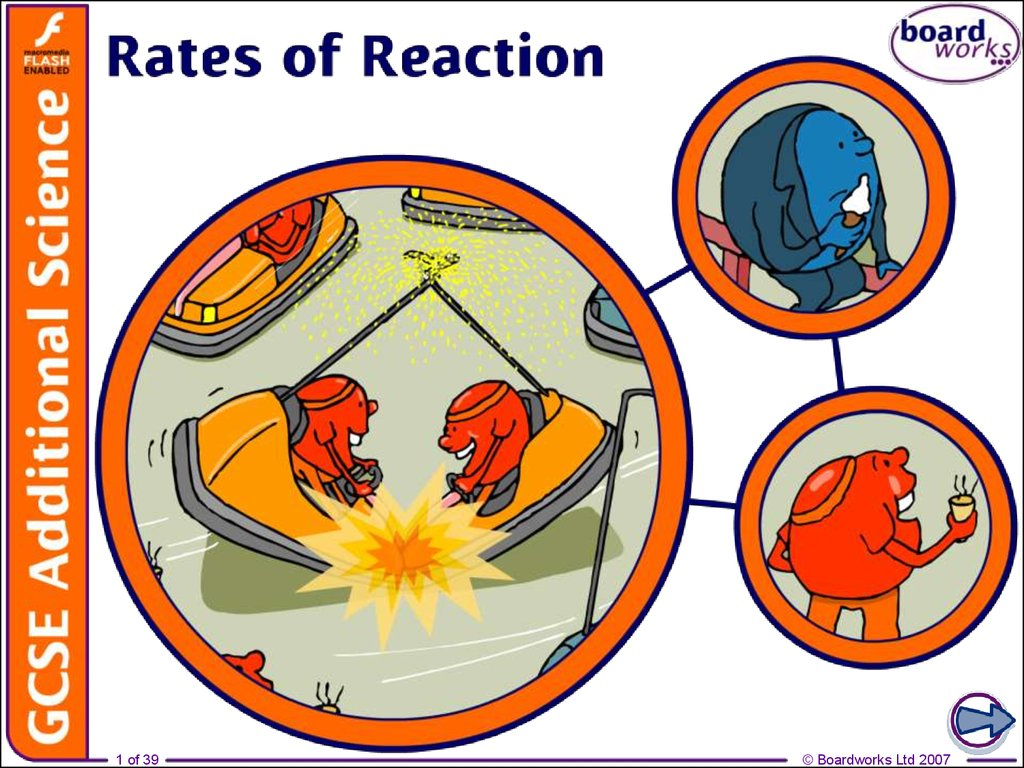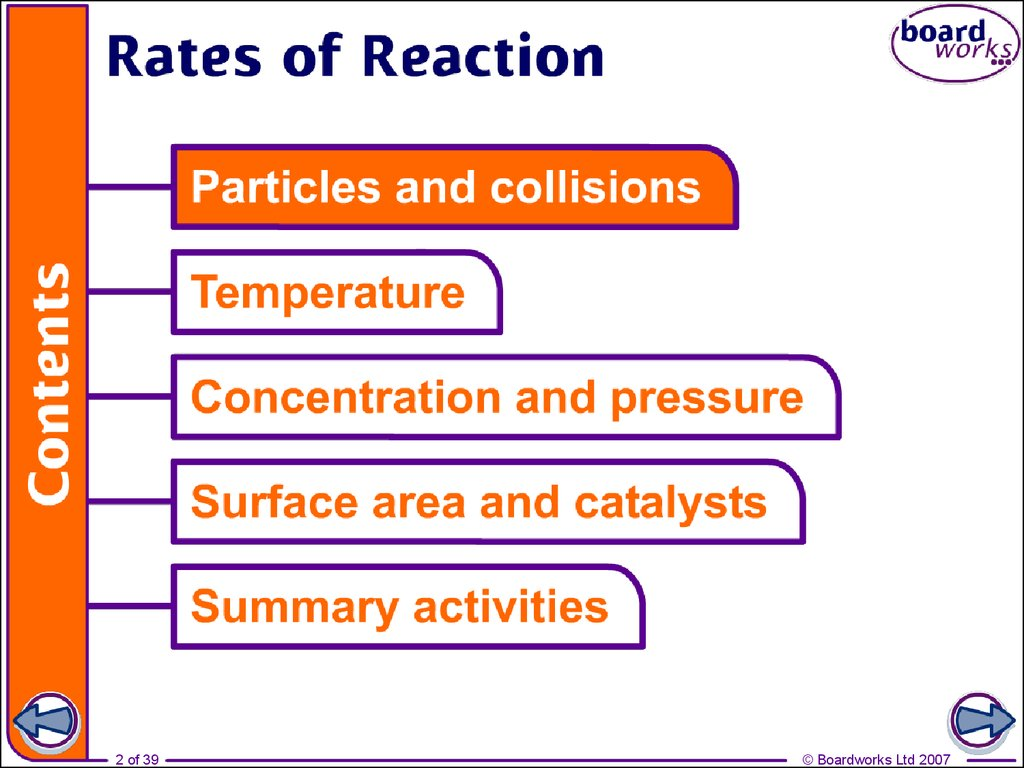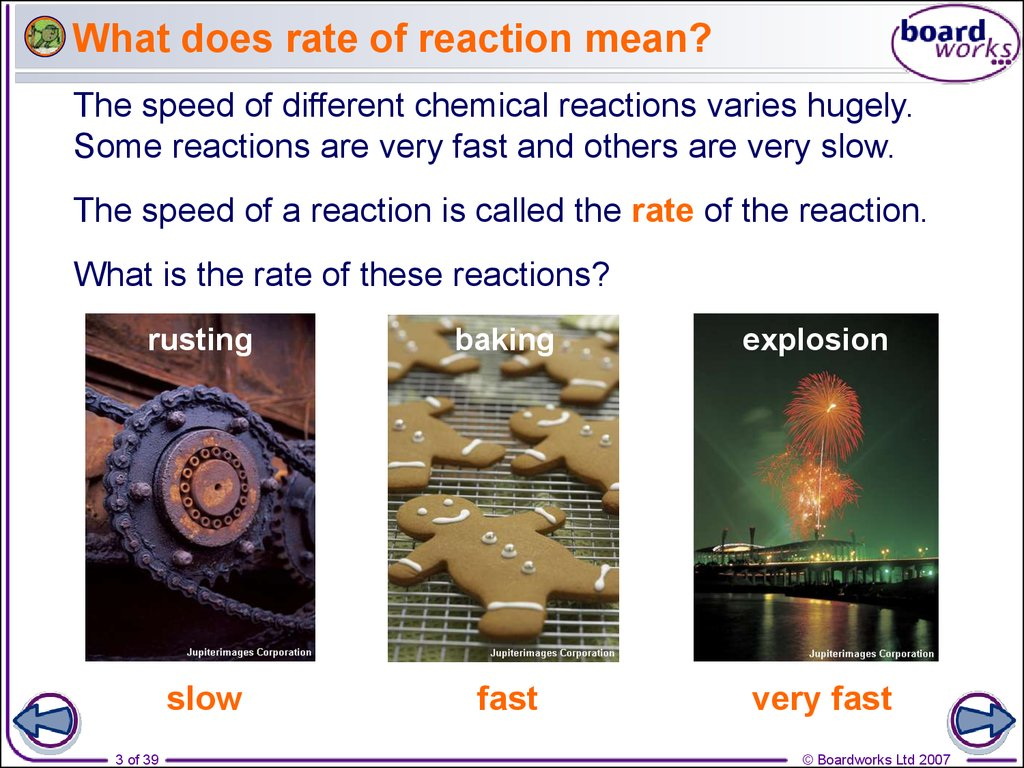Rates of reaction

1 of 39

2 of 39

3. What does rate of reaction mean?

The speed of different chemical reactions varies hugely.
Some reactions are very fast and others are very slow.
The speed of a reaction is called the rate of the reaction.
What is the rate of these reactions?
rusting
baking
explosion
slow
fast
very fast
3 of 39

4. Rates of reaction

Why are some reactions faster than others?
4 of 39

5. Reactions, particles and collisions

Reactions take place when particles collide with a
certain amount of energy.
The minimum amount of energy needed for the particles
to react is called the activation energy, and is different
for each reaction.
The rate of a reaction depends on two things:
the frequency of collisions between particles
the energy with which particles collide.
If particles collide with less energy than the activation
energy, they will not react. The particles will just bounce
off each other.
5 of 39

6. Changing the rate of reactions

Anything that increases the number of successful collisions
between reactant particles will speed up a reaction.
What factors affect the rate of reactions?
increased temperature
increased concentration of
dissolved reactants, and increased
pressure of gaseous reactants
increased surface area of solid
reactants
use of a catalyst.
6 of 39

7. Slower and slower!

Reactions do not proceed at a steady rate. They start off at a
certain speed, then get slower and slower until they stop.
As the reaction progresses, the concentration of reactants
decreases.
This reduces the frequency of collisions between particles
and so the reaction slows down.
0%
25%
reactants
product
7 of 39
50%
75%
100%
percentage completion of reaction

8 of 39

9 of 39

10. How can rate of reaction be measured?

Measuring the rate of a reaction means measuring the
change in the amount of a reactant or the amount of a
product.
What can be measured to calculate the rate of reaction
between magnesium and hydrochloric acid?
magnesium
+
hydrochloric
magnesium
acid
chloride
+
hydrogen
The amount of hydrochloric acid used up (cm3/min).
The amount of magnesium chloride produced (g/min).
The amount of hydrogen product (cm3/min).
10 of 39

11. Setting up rate experiments

What equipment is needed to investigate the rate of
hydrogen production?
glass tube
conical
rubber connecter
gas syringe
rubber bung
hydrochloric
acid
magnesium
11 of 39

12. Calculating rate of reaction from graphs

hydrogen produced (cm3)
How can the rate of reaction be calculated from a graph?
70
60
x
50
rate of reaction = y
x
40
30
y
20
10
0
0
10
20
30
40
50
time (seconds)
The gradient of the graph is equal to the initial rate of
reaction at that time
rate of reaction = 45 cm3 rate of reaction = 2.25 cm3/s
20 s
12 of 39

13 of 39

14 of 39

15 of 39

16. Temperature and collisions

How does temperature affect the rate of particle collision?
16 of 39

17. Effect of temperature on rate

The higher the temperature, the faster the rate of a reaction.
In many reactions, a rise in temperature of 10 °C causes the
rate of reaction to approximately double.
Why does increased temperature
increase the rate of reaction?
At a higher temperature, particles
have more energy. This means
they move faster and are more
likely to collide with other particles.
When the particles collide, they
do so with more energy, and so
the number of successful
collisions increases.
17 of 39

18 of 39

19. Temperature and batteries

Why are batteries more likely to rundown more quickly in
cold weather?
At low temperatures the
reaction that generates the
electric current proceeds
more slowly than at higher
temperatures.
This means batteries are
less likely to deliver enough
current to meet demand.
19 of 39

20. How does temperature affect rate?

The reaction between sodium thiosulfate and hydrochloric
acid produces sulfur.
sodium
sodium
hydrochloric
chloride
thiosulfate +
acid
Na2S2O3
(aq)
+
2HCl
(aq)
2NaCl
(aq)
+
sulfur
dioxide
+
SO2
(g)
+
sulfur
+
S
(s)
+ water
+
H2O
(l)
Sulfur is solid and so it turns the solution cloudy.
How can this fact be used to measure the effect of
temperature on rate of reaction?
20 of 39

21 of 39

22 of 39

23. Effect of concentration on rate of reaction

The higher the concentration of a dissolved reactant, the
faster the rate of a reaction.
Why does increased concentration increase the rate of
reaction?
At a higher concentration, there are more particles in the
same amount of space. This means that the particles are
more likely to collide and therefore more likely to react.
lower concentration
23 of 39
higher concentration

24 of 39

25 of 39

26. Effect of pressure on rate of reaction

Why does increasing the pressure of gaseous reactants
increase the rate of reaction?
As the pressure increases, the space in which the gas
particles are moving becomes smaller.
The gas particles become closer together, increasing the
frequency of collisions. This means that the particles are more
likely to react.
lower pressure
26 of 39
higher pressure

27 of 39

28. Effect of surface area on rate of reaction

Any reaction involving a solid can only take place at the
surface of the solid.
If the solid is split into several pieces, the surface area
increases. What effect will this have on rate of reaction?
low surface area
high surface area
This means that there is an increased area for the reactant
particles to collide with.
The smaller the pieces, the larger the surface area. This
means more collisions and a greater chance of reaction.
28 of 39

29 of 39

30. Reaction between a carbonate and acid

Marble chips are made of calcium carbonate. They react
with hydrochloric acid to produce carbon dioxide.
calcium
carbonate
CaCO3
(aq)
+
+
hydrochloric
acid
2HCl
(aq)
calcium
chloride
CaCl2
(aq)
+
water
+
H2O
(aq)
+
carbon
dioxide
+
CO2
(g)
The effect of increasing surface area on the rate of reaction
can be measured by comparing how quickly the mass of the
reactants decreases using marble chips of different sizes.
30 of 39

31 of 39

32. What are catalysts?

Catalysts are substances that change the rate of a reaction
without being used up in the reaction.
Catalysts never produce more product – they just
produce the same amount more quickly.
energy (kJ)
Ea without
catalyst
Different catalysts work in
different ways, but most
lower the reaction’s
activation energy (Ea).
Ea with
catalyst
reaction (time)
32 of 39

33. Everyday catalysts

Many catalysts are transition metals or their compounds.
For example:
Nickel is a catalyst in the production of margarine
(hydrogenation of vegetable oils).
Iron is a catalyst in the production of ammonia
from nitrogen and hydrogen (the Haber process).
Platinum is a catalyst in the
catalytic converters of car
exhausts. It catalyzes the
conversion of carbon
monoxide and nitrogen
oxide into the less polluting
carbon dioxide and nitrogen.
33 of 39

34. Catalysts in industry

Why are catalysts so important for industry?
quickly, saving time and money.
Catalysts reduce the need for
high temperatures, saving fuel
and reducing pollution.
Catalysts are also essential for living cells. Biological
catalysts are special types of protein called enzymes.
34 of 39

35 of 39

36. Glossary

activation energy – The amount of energy needed to
start a reaction.
catalyst – A substance that increases the rate of a
chemical reaction without being used up.
concentration – The number of molecules of a
substance in a given volume.
enzyme – A biological catalyst.
rate of reaction – The change in the concentration over
a certain period of time.
36 of 39

37 of 39